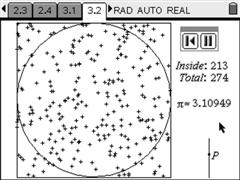PT EN

## Determining Pi by Monte Carlo SimulationA Monte Carlo simulation is a computer program that uses a large number of random events to determine a certain value.

In this case we build a large number of random points inside a square of side 2. Inscribed in that square is a circle of radius 1. The area of the circle is Pi and the area of the square is 4, so each point has a Pi/4 probability of falling inside the circle.

Running the simulation until several hundred points are created we can calculate a numerical approximation of Pi using its results.

This activity includes several questions for the students. After answering them, they can save the document with a new name and transfer it to the teacher's handheld. The teacher can then review their work in his own TI-Nspire.